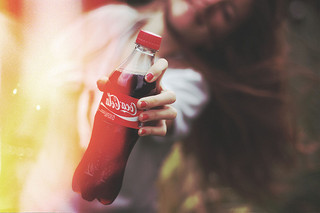# Transformation¶

Note

The image `transform.jpg` used in this docs is taken by Megan Trace, and licensed under CC BY-NC 2.0. It can be found the original photography from Flickr.

## Rotation¶

New in version 0.1.8.

`Image` object provides a simple method to rotate images: `rotate()`. It takes a `degree` which can be 0 to 359. (Actually you can pass 360, 361, or more but it will be the same to 0, 1, or more respectively.)

For example, where the given image `transform.jpg`: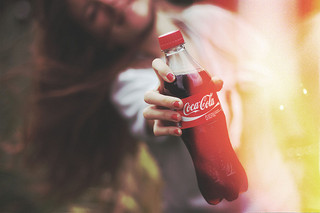The below code makes the image rotated 90° to right:

```from wand.image import Image

with Image(filename='transform.jpg') as image:
with image.clone() as rotated:
rotated.rotate(90)
rotated.save(filename='transform-rotated-90.jpg')
```

The generated image `transform-rotated-90.jpg` looks like: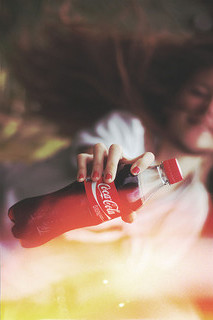If `degree` is not multiples of 90, the optional parameter `background` will help (its default is transparent):

```from wand.color import Color
from wand.image import Image

with Image(filename='transform.jpg') as image:
with image.clone() as rotated:
rotated.rotate(135, background=Color('rgb(229,221,112)'))
rotated.save(filename='transform-rotated-135.jpg')
```

The generated image `transform-rotated-135.jpg` looks like: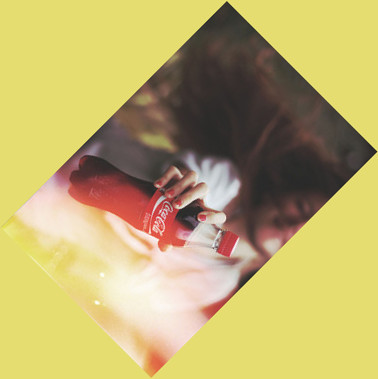## Flip and flop¶

New in version 0.3.0.

You can make a mirror image by reflecting the pixels around the central x- or y-axis. For example, where the given image `transform.jpg`:The following code flips the image using `Image.flip()` method:

```from wand.image import Image

with Image(filename='transform.jpg') as image:
with image.clone() as flipped:
flipped.flip()
flipped.save(filename='transform-flipped.jpg')
```

The image `transform-flipped.jpg` generated by the above code looks like: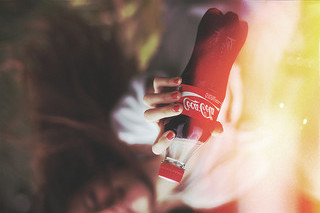As like `flip()`, `flop()` does the same thing except it doesn’t make a vertical mirror image but horizontal:

```from wand.image import Image

with Image(filename='transform.jpg') as image:
with image.clone() as flopped:
flopped.flop()
flopped.save(filename='transform-flopped.jpg')
```

The image `transform-flopped.jpg` generated by the above code looks like: